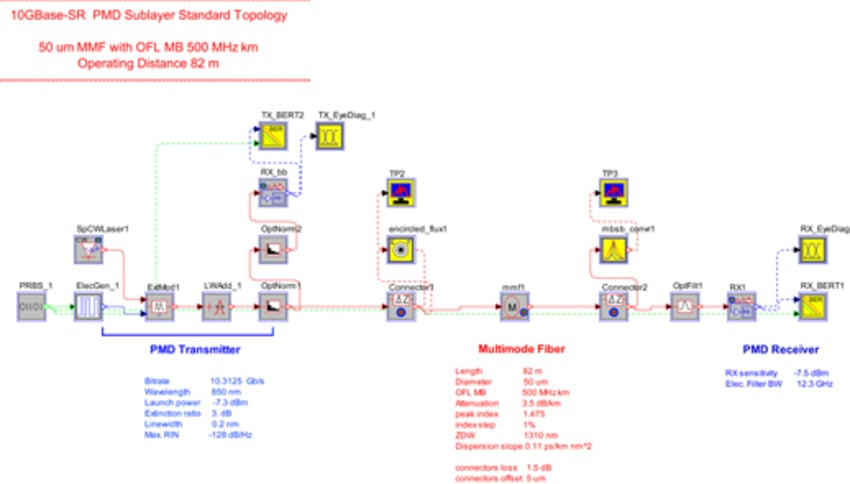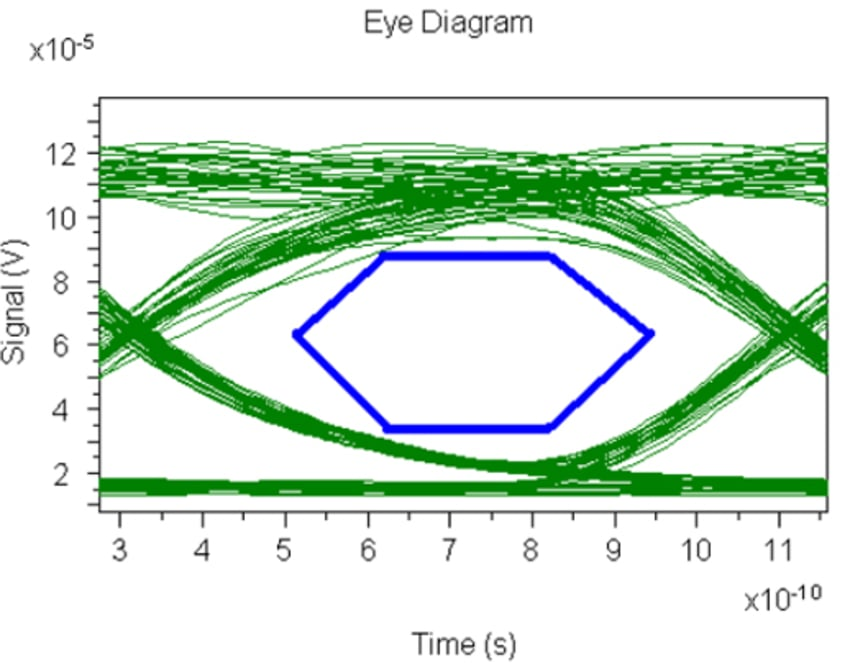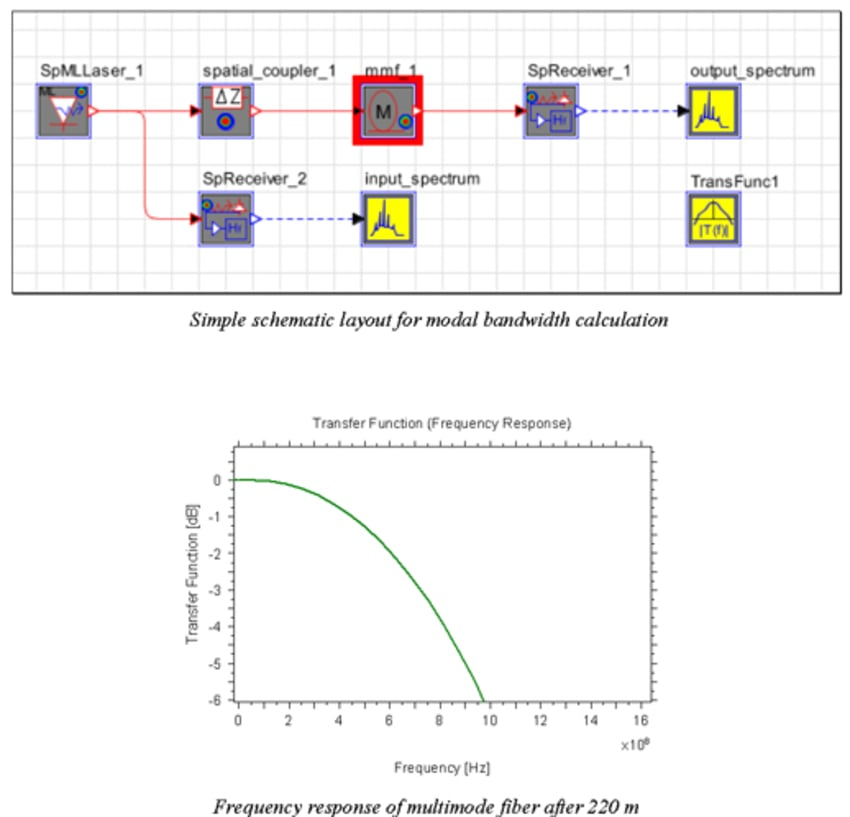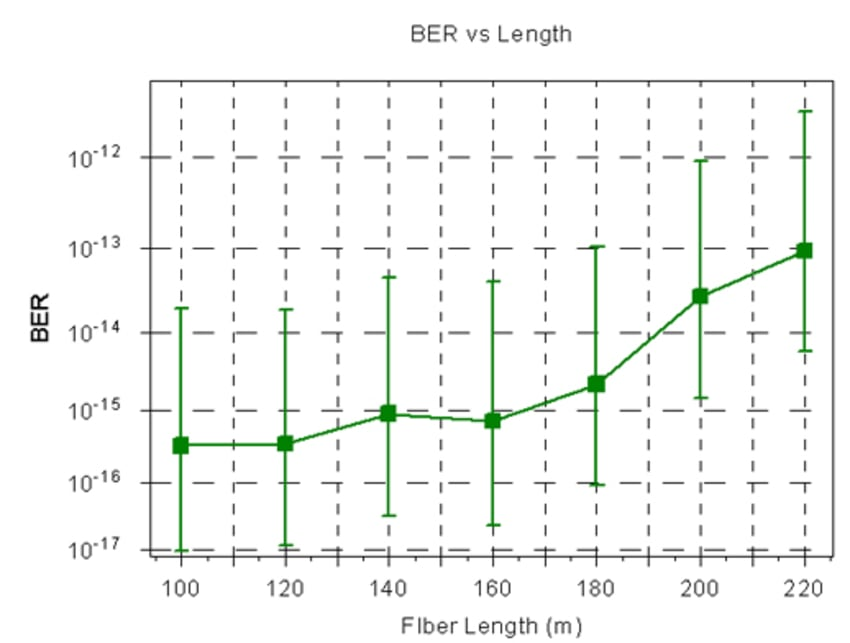# RSoft Application Gallery Note: Gigabit Ethernet Link Design Based on 1000BASE-SX, -LX Standards

## Tools Used: ModeSYS

ModeSYS helps model Gigabit Ethernet Physical Medium Dependent (PMD) sublayer according to IEEE 802.3 Standard. All eight scenarios described in the IEEE 802.3, "Clause 38, Physical Medium Dependent (PMD) sublayer and baseband medium, type 1000BASE -LX (Long Wavelength Laser) and 1000BASE-SX (Short Wavelength Laser)" can be modeled in ModeSYS.

The topology snapshot of 1000Base-SX case with 62.5-micron MMF of 220-m is shown below:The figure below shows simulated transmitter eye diagram along with transmitter eye mask specified in the standard document:The power-law parameter alpha is the one to be optimized to provide required modal bandwidth of the fiber. At alpha = 2 we have an ideal parabolic profile which maximum modal bandwidth. To find out what is the value of parameter alpha required a simple topology for modal bandwidth measurement can be used - see figure below for the layout. The figure also shows a plot of corresponding frequency response out of Transfer Function Analyzer.The receiver eye diagram is shown below:The link performance can then be recalculated at different operating points, such as distance, laser wavelength, launch power, etc. For example, if one wants to re-calculate system performance at different operational distances, he/she can perform a parameter scan over the length of the fiber. For demonstration, here we run a parameter scan over the fiber length ranging from 100 m to 220 m with 20-m steps. Figure below shows resulting BER dependence on the fiber length: